top of page# LSTM Model

Updated: Mar 9

Author: Navneet Kumar

In this blog, we will discuss the Long-Short-Term Memory (LSTM) Recurrent Neural Network, one of the popular deep learning models used in stock market prediction.

The stock market is known for being volatile, dynamic, and nonlinear. Accurate stock price prediction is extremely challenging because of multiple (macro and micro) factors, such as politics, global economic conditions, unexpected events, a company’s financial performance, and so on.

But all of this also means that there’s a lot of data to find patterns in.

Apart from LSTM, we have tried other models also, moving average, Linear Regression,k-nearest Neighbors, Auto ARIMA, and Prophet but none of the models proved to be the right fit for this problem. Moving ahead with the LSTM model, LSTMs are widely used for sequence prediction problems and have proven to be extremely effective. The reason they work so well is that LSTM is able to store past information that is important and forget the information that is not.

Steps to follow:

1. Import necessary library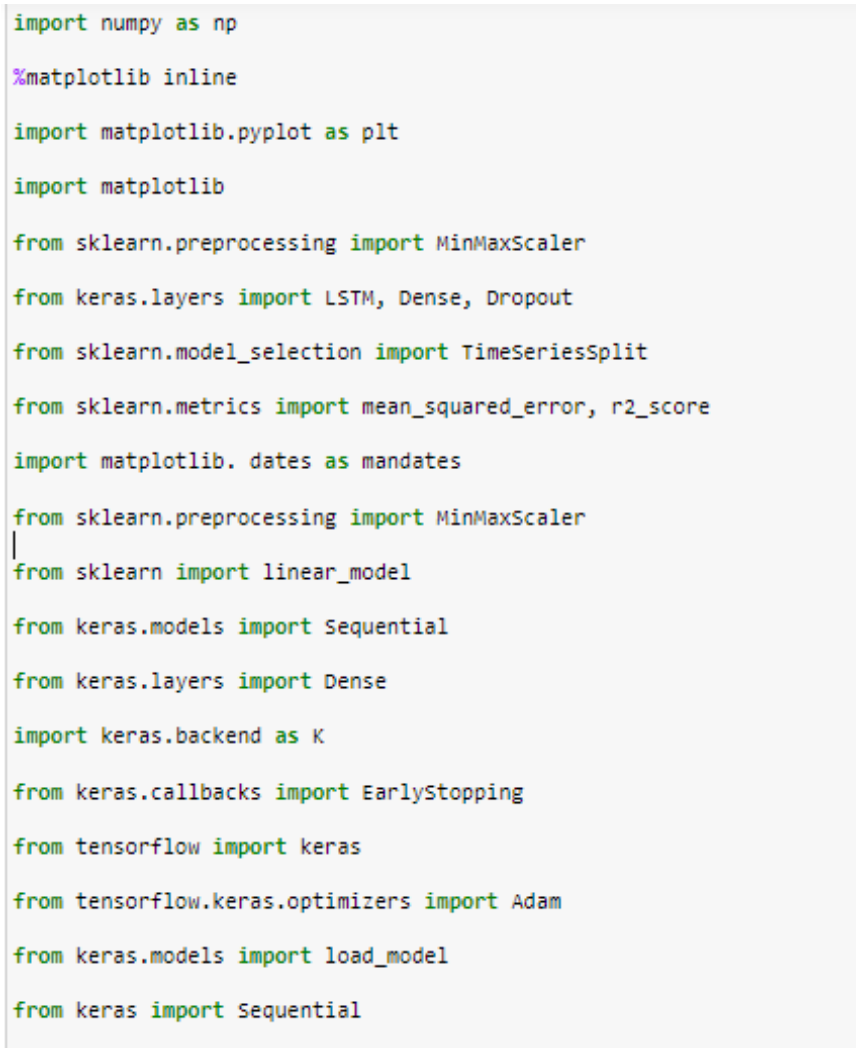2. Read the data; we have read the data from the local drive. We can connect snowflake to python and read the data from the snowflake tables.3. Visualize the data4. Split the data in the test and train set. 80% of data is kept for training, and 20% is for testing, then using the min-max scaler function to normalize the data.5. x_train and y_train are of type list for the further process; we have to convert them into a numpy array6. x_train and y_train are in 2 dimensions, and the LSTM model requires input in 3 dimensions, so we need to add one dimension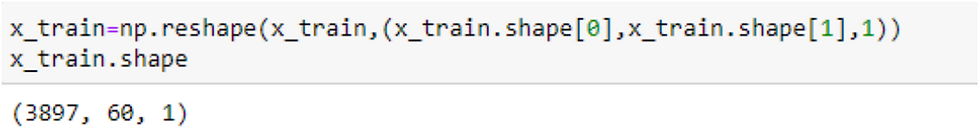7. Next step is to build the LSTM model; we have imported LSTM from keras.layers

This is the step where I faced some challenges; I had to decide how many layers should be perfect for the job, optimize the number of neurons, and drop out.Various techniques can be followed for this; we can use cross-validation. We have used grid search for hyperparameter tuning.

8. Next step is to compile and fit the model, i.e., model training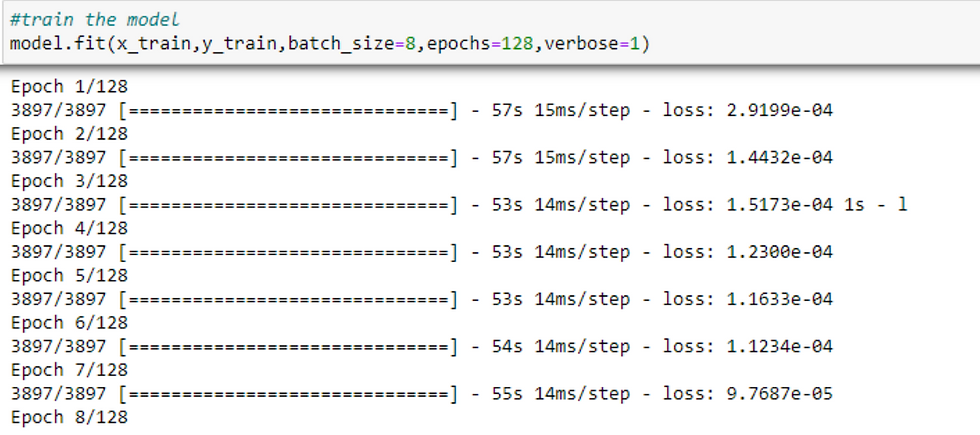9. The next step is to test the accuracy of the test data. For that, we have created a test set just like we did for a train set. We have reshaped x_test as the LSTM model requires input in 3D10. Finally, the step for which we have done this whole process. Making a prediction on the test data and for actual dataHere we predicted the values for the test set and also calculated the RMSE. The more the value close to zero, more is the more accurate. We are getting 51.39; we can make changes to the hyperparameter and can improve the accuracy. But for stock price prediction, we don't get too many accurate models because stock prices not only depend on the close price of the previous days but also on many other factors like the company profit, market condition, company's policies, political decisions, etc.

11. Next, we shall draw a graph for predicted and actual values on test data to check the accuracy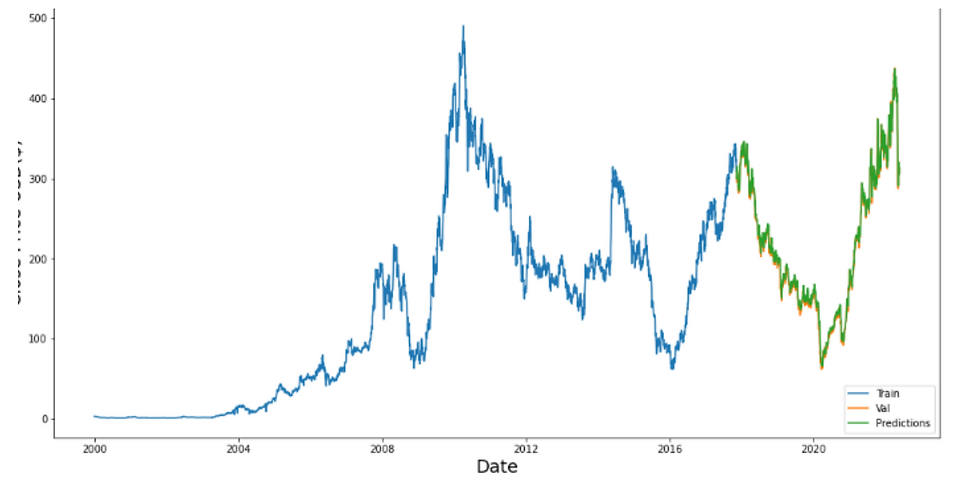So now we have trained the model as the model is working fine on test data. This is the time we check the model for the actual date. For this, we are taking the last 60 days of the snowflake table and feeding it to the mode by the last 60 days. I mean, if we want to predict the close price for today, we need to feed the last 60 days of data, i.e., from yesterday to the last 60 days. As our snowflake tables are up to date, we are taking data from the snowflake table.

12. Some connections are required for this purpose, as all the trained models are stored in an S3 bucket; we had to connect python to the snowflake and also python to snowflake for the last 60 days of data.We have imported all the necessary modules, and a connection to the snowflake has been established. A point to mention is that we are using a snowflake account, so it may be possible by the time this account expires. We have another account for backup.

13. Now, we have connected python to the S3 bucket to load the trained models.14. Now, we will take 60 days of data from snowflake tables and predict the price for the 61st day.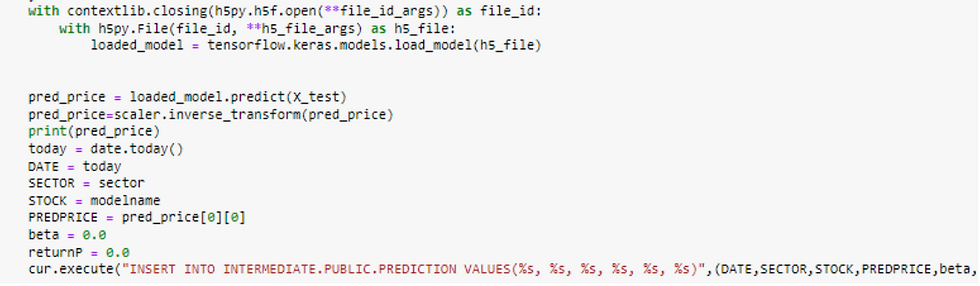This is the end of the code.

The LSTM model can be tuned for various parameters, such as changing the number of LSTM layers, adding dropout value or increasing the number of epochs.